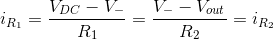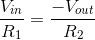# Inverting Amplifier

## Working principle

The inverting amplifier configuration produces an output voltage equal to its input voltage multiplied by a negative ratio of its two resistors:### Formula derivation

Since any ideal op-amp tries to keep its two terminals at an equal voltage, the inverting input of the op-amp (V-) is at the same potential as the non-inverting terminal (V+) and, thus, forms a virtual ground at its node: V- = V+ = 0.

Since the op-amp’s input terminals (V+ and V-) have an infinite input impedance, there is no current flowing into them. Instead, all of the current flowing through R1 also flows through R2:### Experiments

• Vary the input voltage and resistor values and observe the change at the output.
• Change the input voltage to a negative value and verify that the output voltage is now positive (inverted).
• Make R1 and R2 equal to create an inverting buffer. What is the output voltage now in terms of the input voltage?